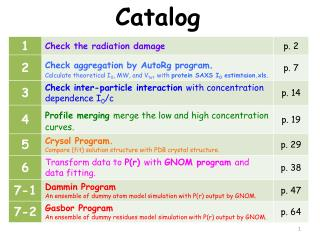DownloadDownload PresentationCatalog

# Catalog

Télécharger la présentation## Catalog

- - - - - - - - - - - - - - - - - - - - - - - - - - - E N D - - - - - - - - - - - - - - - - - - - - - - - - - - -
##### Presentation Transcript

1. Catalog

2. 1. Check the radiation damage

3. 2. Plot all curves in a graph

4. 3. Check the low-q region in the all profiles

5. If all profiles are overlapped well at low-q region, no radiation damage occurs.

6. 2. Check aggregation by AutoRg program. Calculate theoretical I0, MW, and Vtotwith protein SAXS I0 estimtaion.xls.

7. 1. Start program: Primus -> AutoRg

8. 2. Open the input file (file type: txt, dat)

9. 3. In Plot tab, read the raw data.

10. 4. In Guinier tab, check the aggregation and define the qmin before unreliable range.

11. One can calculate theoretical MW, I0, and Vtotwith protein SAXS I0 estimtaion.xls. (spreadsheet edited by Dr. Jeng) 1 3 4 2 5 6

12. 3. Check inter-particle interaction with concentration dependence I0/c

13. 2. Plot all different concentration curves.

14. 3. Set each y and yEr column as I(q)/conc.

15. 4. Profile merging merge the low and high concentration curves.

16. 1. Start program: Primus

17. 2. Click “Tools” to select the low and high concentration data for merging.

18. 3. Click “plot” to plot the two curves.

19. 4. Input the parameters into nBeg (begin point #) and nEnd (end point #) of Data Processing.

20. 4-2. Remove the points of inter-particle interference region and divergence region

21. Initially remove the unreliable points

22. 5. Scale the two curves.

23. 6. Fine tune the eEnd point to make sure that the endpoints are superimposed with the merged curve.

24. 7. Refine the point range for well-merge .

25. 5. Crysol Program Compare (fit) solution structure with PDB crystal structure.

26. 1. Enter your option <0>: 

27. 2. Select the PDB file (protein data bank)

28. 3. Maximum order of harmonics <15>:  Order of Fibonacci grid <17>:  Maximum s value <0.5>:  Number of points <51>:  Account for explicit hydrogens <no>:  Fit the experimental curve <yes>: 

29. 4. Enter data file (experimental dat file)

30. 5. Subtract constant <no>:  Angular units in the input file <1>:  Electron density of the solvent <0.334>:  Plot the fit <yes>:  Another set of parameters <no>:  Press “ ” to terminate the program

31. 6. Plot the 1st (experimental scattering vector) and 2nd (theoretical intensity in solution) columns of fit file. fitted solution envelope of native cyt. c

32. 7. Open the log file to read the experimental and theoretical Rg values.

33. 6. Transform data to P(r) with GNOM program and data fitting.

34. 1. Input data, first file : (select the file)

35. 2. Input Output file[ gnom.out ] : filename.out

36. 3. • No of start points to skip [ 0 ] : • Input data, second file [ none ] :  • No of end points to omit [ 0 ] :  • Angular scale (1/2/3/4) [ 1 ] :  • Plot input data (Y/N) [ Yes ] : 

37. 4. • File containing expert parameters [ none ] :  • Kernel already calculated (Y/N) [ No ] :  • Type of system (0/1/2/3/4/5/6) [ 0 ] :  • Zero condition at r=rmin (Y/N) [ Yes ] :  • Zero condition at r=rmax (Y/N) [ Yes ] :

38. 5. Rmaxfor evaluating p(r) : (input a proper value) 

39. 6. • Number of points in real space [ 101 ] :  • Kernel-storage file name [ kern.bin ] :  • Experimental setup (0/1/2) [ 0 ] :  • Initial ALPHA [ 0.0 ] : 

40. 7. Plot results (Y/N) [ Yes ] : 

41. 8. GNOM fit shows probability distribution function P(r)

42. 7-1. DamminProgram An ensemble of dummy atom model simulation with P(r) output by GNOM.

43. 1. Select the Mode: <[F]>ast, [S]low, [J]ag, [K]eep, [E]xpert < Fast >: 

44. 3. Input data, GNOM output file name < .out >: filename 